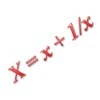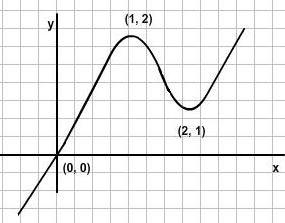#### You may also like### The Why and How of Substitution

Step back and reflect! This article reviews techniques such as substitution and change of coordinates which enable us to exploit underlying structures to crack problems.### Polynomial Interpolation

Can you fit polynomials through these points?

# Curve Fitter

##### Age 16 to 18 Challenge Level:

I wish to find a function which passes through the origin and only has turning points at $(1, 2)$ and $(2,1)$, as in the following sketch:What sorts of equations would be good candidates for having a graph of this form? Can you definitely rule out certain types of equation from having a graph like this?

Although this curve might look like a cubic equation, it actually cannot be a cubic equation. Prove that this is the case.

Next, can you explicity find an equation which exactly satisfies these conditions and looks similar to the graph shown (i.e. with no other turning points anywhere and the same asymptotic behaviour)? How might you assess the effectiveness of the 'similarity'?